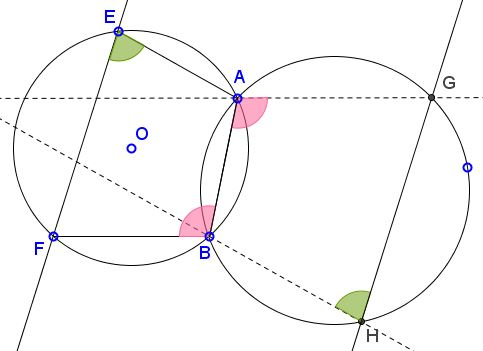# Reim's Similar Coins II

### Problem

Let two circles cross at points $A$ and $B;$ $E$ and $F$ are two points on one of the circles, $G$ and $H$ on the other. Assume $AG\parallel FB$ and $BH\parallel EA.$Then $GH\parallel EF.$

### Solution

Chasing angles leads to a simple solution.As a pair of vertical angles in parallel lines $(AG\parallel FB),)$ $\angle ABF=\angle BAG.$ Further, since quadrilaterals $ABFE$ and $ABHG$ are cyclic, $\angle ABF+\angle AEF=180^{\circ}$ and also $\angle BAG+\angle BHG=180^{\circ},$ implying $\angle AEF=\angle BHG.$ These two angles have one pair of sides parallel $(BH\parallel EA.)$ So their other sides are also parallel, $GH\parallel EF.$

### Converse 1

Given a cyclic quadrilateral $ABFE$ and points $G$ and $H$ such that $AG||FB$ and $BH\parallel EA.$ If $GH\parallel EF,$ then quadrilateral $ABHG$ is cyclic.### Converse 2

Let two circles cross at points $A$ and $B;$ $E$ and $F$ are two points on one of the circles; $AG||FB$ whereas chord $GH$ is parallel to $EF.$Then $BH\parallel EA.$

For, if $BH'||AE,$ with $H'$ on the second circle, then $GH'\parallel EF\parallel GH,$ implying that $H=H'.$

### Acknowledgment

I confess to not knowing the reason for the theorem designation. There is a companion theorem under the same attribution. I came across this one in an article by Jean-Louis Ayme where he referred to it as "Le théorème des moniennes semblables de Reim" which both I and google had a difficulty translating. It looks to me like "Reim's similar coins" might be a good fit, but I am not sure.

### Chasing Inscribed Angles